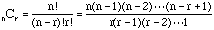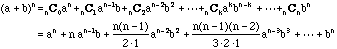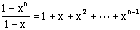Subject: Math: Binomial Thrm. Name: Angela Who is asking: Student Level: Secondary Question: The full question is this: Isaac Newton generalized the Binomial Theorem to rational exponents. That is, he derived series of expansions for such expressions as (x+y)-3 (x+y)2/3 (x+y)5/6 What did Newton find? What are the first four terms of the series expansions of binomials above? How can this extended Binomial Thrm. be used to aid in calculations? First, I expanded the (x+y)-3 and got: 1/(x3+3xy+3xy2+y3) But I don't know how to expand the last two. I tried to and got: (x2 + 2xy + y2)/(cubed root(x+y)) ??? I don't know if I did those right. I don't understand how this relates to the Bionomial Thrm. and what Newton found. Please help me! I'm desperate!! Thank you so much! Hi Angela For n a positive integer andthe Binomial Theorem states thatNewton generalized this for n not a positive integer. The easiest example comes from looking at the identitywhich is valid provided x is not 1. To see that this is valid multiply both sides by 1-x.    Now what happens as n tends to infinity? If -1

Go to Math Central